#### Normal contact stiffness

A node-to-face contact element consists of a slave node connected to a master face (cf. Figure 128). Therefore, it consists of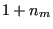nodes, where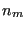is the number of nodes belonging to the master face. The stiffness matrix of a finite element is the derivative of the internal forces in each of the nodes w.r.t. the displacements of each of the nodes. Therefore, we need to determine the internal force in the nodes.

Denoting the position of the slave node by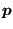and the position of the projection onto the master face by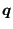, the vector connecting both satisfies: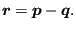(204)

The clearance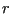at this position can be described by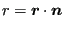(205)

where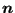is the local normal on the master face. Denoting the nodes belonging to the master face by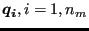and the local coordinates within the face by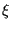and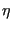, one can write: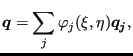(206)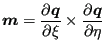(207)

and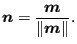(208)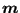is the Jacobian vector on the surface. The internal force on node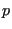is now given by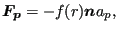(209)

where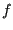is the pressure versus clearance function selected by the user and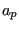is the slave area for which nodeis representative. If the slave node belongs to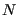contact slave faces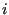with area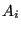, this area may be calculated as: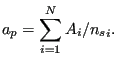(210)

The minus sign in Equation (209) stems from the fact that the internal force is minus the external force (the external force is the force the master face exerts on the slave node). Replacing the normal in Equation (209) by the Jacobian vector devided by its norm and taking the derivative w.r.t.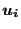, wherecan be the slave node or any node belonging to the master face one obtains: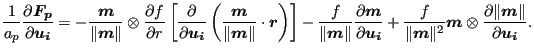(211)

Since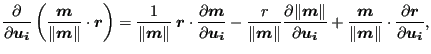(212)

the above equation can be rewritten as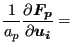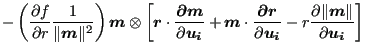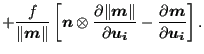(213)

Consequently, the derivatives which are left to be determined are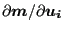,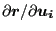and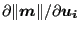.

The derivative ofis obtained by considering Equation (207), which can also be written as: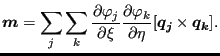(214)

Derivation yields (notice thatandare a function of, and that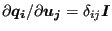) :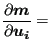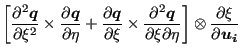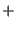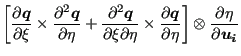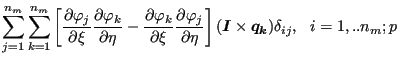(215)

The derivatives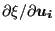and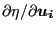on the right hand side are unknown and will be determined later on. They represent the change ofandwhenever any of theis changed, k being the slave node or any of the nodes belonging to the master face. Recall that the value ofandis obtained by orthogonal projection of the slave node on the master face.

Combining Equations (204) and (206) to obtain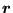, the derivative w.r.t.can be written as: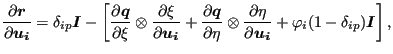(216)

whererepresents the slave node.

Finally, the derivative of the norm of a vector can be written as a function of the derivative of the vector itself: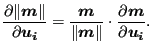(217)

The only derivatives left to determine are the derivatives ofandw.r.t.. Requiring thatis the orthogonal projection ofonto the master face is equivalent to expressing that the connecting vectoris orthogonal to the vectors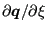and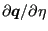, which are tangent to the master surface.

Now,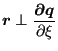(218)

can be rewritten as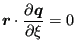(219)

or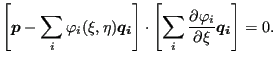(220)

Differentation of the above expression leads to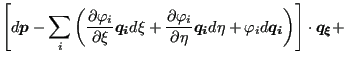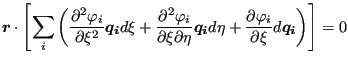(221)

where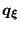is the derivative ofw.r.t.. The above equation is equivalent to: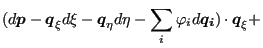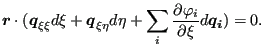(222)

One finally arrives at: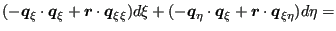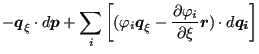(223)

and similarly for the tangent in-direction: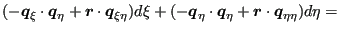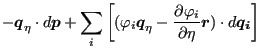(224)

From this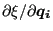,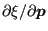and so on can be determined. Indeed, suppose that all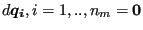and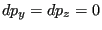. Then, the right hand side of the above equations reduces to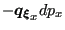and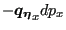and one ends up with two equations in the two unknowns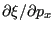and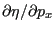. Onceis determined one automatically obtains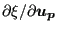since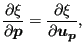(225)

and similarly for the other derivatives. This concludes the derivation of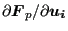.

Since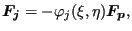(226)

one obtains: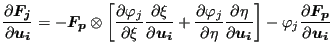(227)

for the derivatives of the forces in the master nodes.